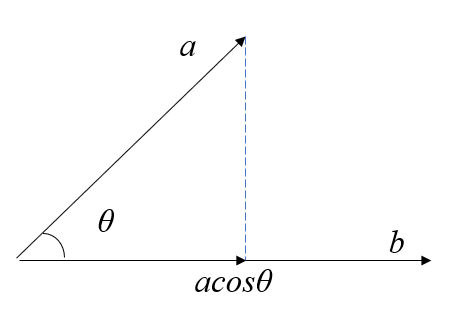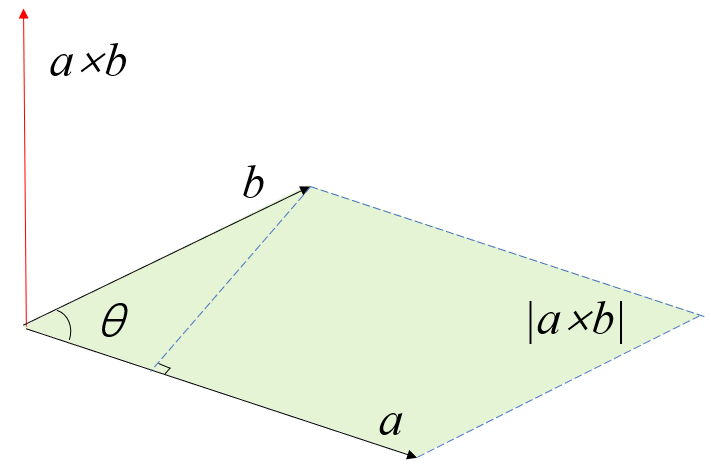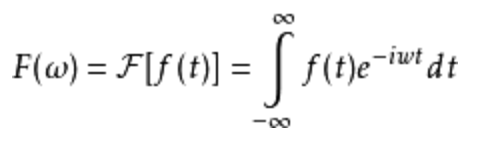• 2017-11-12 22:36:10

### 如何判断向量正交：

内积：对应位置相乘再求和，是内积

卷积：加上滑动窗口

判断向量是否正交：

两个向量正交：求其内积，看是否为0，若为零，则正交。

在空间上向量垂直就正交。

例子：a=(1,1,0),b=(1,-1,0) ，则内积(a,b)=1*1+1*(-1)+0*0=0，所以a，b正交。

正交向量

“正交向量”是一个数学术语，指点积为零的两个或多个向量

换句话说， 两个向量正交意味着它们是相互垂直的。若向量α与β正交，则记为α⊥β

如何求解正交矩阵：

https://jingyan.baidu.com/article/9faa72318364a7473c28cbe9.html

## 正交矩阵

正交矩阵是实数特殊化的酉矩阵，因此总是正规矩阵。尽管我们在这里只考虑实数矩阵，这个定义可用于其元素来自任何域的矩阵。正交矩阵毕竟是从内积自然引出的，对于复数的矩阵这导致了归一要求。

## 定义

定义 1
如果：AA'=E（E为单位矩阵，A'表示“矩阵A的转置矩阵”。）或A′A=E，则n阶实矩阵 A称为正交矩阵， 若A为正交阵，则满足以下条件:
1) A 是正交矩阵
3) A′是正交矩阵
4) A的各行是单位向量且两两正交
5) A的各列是单位向量且两两正交
6) (Ax,Ay)=(x,y) x,y∈R
正交矩阵通常用字母Q表示。
举例：A=[r11 r12 r13;r21 r22 r23;r31 r32 r33]
则有：
r11^2+r12^2+r13^2=r21^2+r22^2+r23^2=r31^2+r32^2+r33^2=1
r11*r12+r21*r22+r31*r32=0等性质

正交方阵是欧氏空间中标准正交基到标准正交基的过渡矩阵。
在 矩阵论中， 实数 正交矩阵方块矩阵 Q，它的 转置矩阵是它的 逆矩阵:
，如果正交矩阵的 行列式为 +1，则我们称之为 特殊正交矩阵:

## 概述

要看出与内积的联系，考虑在  n 维实数 内积空间中的关于正交基写出的向量 vv 的长度的平方是 vv。如果矩阵形式为 Q v 的线性变换保持了向量长度，则
所以有限维线性 等距同构，比如 旋转反射和它们的组合，都产生正交矩阵。反过来也成立: 正交矩阵蕴涵了正交变换。但是， 线性代数包括了在既不是有限维的也不是同样维度的空间之间的 正交变换，它们没有等价的正交矩阵。
有多种原由使正交矩阵对理论和实践是重要的。 n× n 正交矩阵形成了一个 ，即指示为 O( n) 的 正交群，它和它的子群广泛的用在数学和物理科学中。例如，分子的 点群O(3) 的子群。因为浮点版本的正交矩阵有有利的性质，它们是字数值线性代数中很多算法比如 QR分解的关键，通过适当的规范化， 离散余弦变换 (用于 MP3 压缩)可用正交矩阵表示。

## 例子

下面是一些小正交矩阵的例子和可能的解释。
恒等变换。 旋转 16.26°。 针对  x 轴反射。 旋转反演(rotoinversion): 轴 (0,-3/5,4/5)，角度90°。 置换坐标轴。

## 基本构造

### 低维度

最简单的正交矩阵是 1×1 矩阵  和 [−1]，它们可分别解释为恒等和实数线针对原点的反射。
如下形式的 2×2 矩阵
它的正交性要求满足三个方程
在考虑第一个方程时，不丢失一般性而设  p = cos θ,  q = sin θ；因此要么  t = − q, u = p 要么 t =  qu = − p。我们可以解释第一种情况为旋转 θ(θ = 0 是单位矩阵)，第二个解释为针对在角 θ/2 的直线的反射。
旋转 反射 在 45°的反射对换  x 和  y；它是 置换矩阵，在每列和每行带有一个单一的 1(其他都是 0):
单位矩阵也是置换矩阵。
反射是它自己的逆，这蕴涵了反射矩阵是 对称的(等于它的转置矩阵)也是正交的。两个旋转矩阵的积是一个旋转矩阵，两个反射矩阵的积也是旋转矩阵。

### 更高维度

不管维度，总是可能把正交矩阵按纯旋转与否来分类，但是对于 3×3 矩阵和更高维度矩阵要比反射复杂多了。例如，
和 表示通过原点的 反演和关于 z 轴的旋转反演(逆时针旋转90°后针对 x- y平面反射，或逆时针旋转 270°后对原点反演)。
旋转也变得更加复杂；它们不再由一个角来刻画，并可能影响多于一个平面子空间。尽管经常以一个轴和角来描述 3×3 旋转矩阵，在这个维度旋转轴的存在是偶然的性质而不适用于其他维度。
但是，我们有了一般适用的基本建造板块如置换、反射、和旋转。

### 基本变换

最基本的置换是换位(transposition)，通过交换单位矩阵的两行得到。任何  n× n 置换矩阵都可以构造为最多  n−1 次换位的积。 构造自非零向量  v 的 Householder反射为
这里的分子是对称矩阵，而分母是  v 的平方量的一个数。这是在垂直于  v 的超平面上的反射(取负平行于 v 任何向量分量)。如果 v 是单位向量，则 Q =  I−2 vv 就足够了。Householder 反射典型的用于同时置零一列的较低部分。任何 n× n 正交矩阵都可以构造为最多 n 次这种反射的积。
Givens旋转作用于由两个坐标轴所生成的二维(平面)子空间上，按选定角度旋转。它典型的用来置零一个单一的次对角线元素(subdiagonal entry)。任何 n× n 的旋转矩阵都可以构造为最多 n( n−1)/2 次这种旋转的积。在 3x3 矩阵的情况下，三个这种旋转就足够了；并且通过固定这个序列，我们可以用经常叫做 欧拉角的三个角来(尽管不唯一)描述所有 3×3 旋转矩阵。
雅可比旋转有同 Givens 旋转一样的形式，但是被用做 相似变换，选择来置零 2×2 子矩阵的两个远离对角元素(off-diagonal entry)。

## 性质

### 矩阵性质

实数方块矩阵是正交的，当且仅当它的列形成了带有普通欧几里得 点积欧几里得空间 R 的正交规范基，它为真当且仅当它的行形成 R 的正交基。假设带有正交(非正交规范)列的矩阵叫正交矩阵可能是诱人的，但是这种矩阵没有特殊价值而没有特殊名字；他们只是 MM = DD 是 对角矩阵
任何正交矩阵的行列式是 +1 或 −1。这可从关于行列式的如下基本事实得出:
反过来不是真的；有 +1 行列式不保证正交性，即使带有正交列，可由下列反例证实。
对于置换矩阵，行列式是 +1 还是 −1 匹配置换是偶还是奇的 标志，行列式是行的交替函数。
比行列式限制更强的是正交矩阵总可以是在复数上可对角化来展示特征值的完全的集合，它们全都必须有(复数)绝对值 1。

### 群性质

正交矩阵的逆是正交的，两个正交矩阵的积是正交的。事实上，所有  n× n 正交矩阵的集合满足群的所有公理。它是  n( n−1)/2 维的 紧致 李群，叫做正交群并指示为 O( n)。
行列式为 +1 的正交矩阵形成了路径连通的子群指标为 2 的  O( n正规子群，叫做旋转的特殊正交群  SO( n)。 商群 O( n)/ SO( n) 同构于 O(1)，带有依据行列式选择 [+1] 或 [−1] 的投影映射。带有行列式 −1 的正交矩阵不包括单位矩阵，所以不形成子群而只是 陪集；它也是(分离的)连通的。所以每个正交群被分为两个部分；因为投影映射 分裂O( n) 是  SO( n) 与  O(1)的 半直积。用实用术语说，一个相当的陈述是任何正交矩阵可以通过采用一个旋转矩阵并可能取负它的一列来生成，如我们在 2×2 矩阵中看到的。如果 n 是奇数，则半直积实际上是 直积，任何正交矩阵可以通过采用一个旋转矩阵并可能取负它的所有列来生成。
现在考虑 ( n+1)×( n+1) 右底元素等于 1 的正交矩阵。最后一列(和最后一行)的余下元素必须是零，而任何两个这种矩阵的积有同样的形式。余下的矩阵是 n× n 正交矩阵；因此 O( n) 是 O( n+1) (和所有更高维群)的子群。
因为 Householder 正交矩阵形式的基本反射可把任何正交矩阵简约成这种约束形式，一系列的这种反射可以把任何正交矩阵变回单位矩阵；因此正交群是反射群。最后一列可以被固定为任何单位向量，并且每种选择给出不同的 O( n) 在 O( n+1) 中的复本；以这种方式 O( n+1) 是在单位球 S 与纤维  O( n) 上的
类似的， SO( n) 是  SO( n+1) 的子群；任何特定正交矩阵可以使用类似过程通过 Givens 平面旋转来生成。丛结构持续: SO( n) ↪ SO( n+1) → S。一个单一旋转可以在最后一列的第一行生成一个零，而 n−1 次旋转序列将置零 n× n 旋转矩阵的除了最后一列的最后一行的所有元素。因为平面是固定的，每次旋转只有一个自由度，就是它的角度。通过归纳， SO( n) 因此有
自由度， O( n) 也是。
置换矩阵简单一些；它们不形成李群，只是一个有限群， n! 次 对称群 Sn。通过同类的讨论， Sn 是 Sn+1 的子群。偶置换生成行列式 +1 的置换矩阵的子群， n!/2 次交错群。

### 规范形式

更广泛的说，任何正交矩阵的效果分离到在正交二维空间上的独立动作。就是说，如果  Q 是狭义正交的，则你可以找到(旋转)改变基的一个正交矩阵  P，把  Q 带回到分块对角形式:
( n 偶数)， ( n 奇数)。 这里的矩阵  R1,..., Rk 是 2×2 旋转矩阵，而余下的元素是零。作为例外，一个旋转块可以是对角的， ± I。因此如果需要的话取负一列，并注意 2×2 反射可对角化为 +1 和 −1，任何正交矩阵可变为如下形式
, 矩阵  R1,…, Rk 给出位于 复平面中单位圆上的特征值的共轭对；所以这个分解复合确定所有带有绝对值 1 的特征值。如果 n 是奇数，至少有一个实数特征值 +1 或 −1；对于 3×3 旋转，关联着 +1 的特征向量是旋转轴。

## 数值线性代数

### 利益

数值分析自然的利用了正交矩阵的很多数值线性代数的性质。例如，经常需要计算空间的正交基，或基的正交变更；二者都采用了正交矩阵的形式。有行列式 ±1 和所有模为 1 的特征值是对数值稳定性非常有利的。 一个蕴涵是 条件数为 1 (这是极小的)，所以在乘以正交矩阵的时候错误不放大。很多算法为此使用正交矩阵如 Householder反射和 Givens旋转。有帮助的不只是正交矩阵是可逆的，还有它的逆矩阵本质上是免花费的，只需要对换索引(下标)。
置换是很多算法成功的根本，包括有局部定支点(partial pivoting)的运算繁重的 高斯消去法(这里的置换用来定支点)。但是它们很少明显作为矩阵出现；它们的特殊形式允许更有限的表示，比如 n 个索引的列表。
同样的，使用 Householder 和 Givens 矩阵的算法典型的使用特殊方法的乘法和存储。例如，Givens 旋转只影响它所乘的矩阵的两行，替代完全的 n 次的 矩阵乘法为更有效的 n 次运算。在使用这些反射和旋转向矩阵介入零的时候，腾出的空间足够存储充足的数据来重生成这个变换。

### 分解

一些重要的 矩阵分解(Golub & Van Loan, 1996)涉及到了正交矩阵，包括:
QR分解  M =  QRQ 正交， R 上三角。  奇异值分解  M =  UΣVU 和  V 正交， Σ 非负对角。 谱分解 S = QΛQ, S 对称， Q 正交， Λ 对角。 极分解  M = QS, Q 正交， S 对称非负确定。

更多相关内容
• 2 向量正交 3 函数的正交 4 函数正交的意义 1 向量内积和外积 1.1 向量内积(点乘) 假设 a = [a1,a2,...,an], b = [b1,b2,...,bn]； a与b的内积为 a·b = a1·b1+a2·b2+...+a3·b3 = |a||b|cosθ； ...

目录

1 向量内积和外积

1.1 向量内积(点乘)

1.2 向量外积(差乘)

2 向量的正交

3 函数的正交

4 函数正交的意义

## 1 向量内积和外积

### 1.1 向量内积(点乘)

假设  a = [a1,a2,...,an],  b = [b1,b2,...,bn]；

则a与b的内积为  a·b = a1·b1+a2·b2+...+a3·b3 = |a||b|cosθ；点成的结果是  标量

几何意义：b向量在a向量方向上的投影

作用：用来证明两个向量是否正交。则表示，θ为90°（的奇数倍），即表示两个非零向量垂直（即正交）。

### 1.2 向量外积(差乘)

假设  a = [a1,a2,...,an],  b = [b1,b2,...,bn]；对应单位向量是  [e1,e2,...,en];

则a与b的外积

大小：  |a ×b| = |a|·|b|·sin<a,b>.

方向：法向量。 根据右手法则确定，就是手掌立在ab所在平面的向量a上，掌心由a转向b的过程中，大拇指的方向是外积方向。（∴方向与乘积的顺序有关点成的结果是  向量

几何意义：大小是以a、b为相邻边的平行四边形围成的面积；方向是法向量。

作用：用来证明两个向量是否平行。

## 2 向量的正交

正交 线性代数的概念，是垂直这一直观概念的推广。作为一个形容词，只有在一个确定的内积空间中才有意义。若内积空间中两向量内积为0，则称它们是正交的。如果能够定义向量间的夹角，则正交可以直观的理解为垂直。物理中：运动的独立性，也可以用正交来解释。[百度百科：正交]

向量正交

1. 1、从几何上来理解

如果是零向量，它与任何向量正交。如果非零向量之间正交，则它们之间是垂直的，可以简单理解为向量之间的夹角为90°，或者其中一个向量在另一个向量上的投影长度为0（变成一个点）

2. 2、从代数上来理解

所有同维向量构成一个向量空间，正交的向量之间，满足内积为0。简单来说，就是向量各分量之间相乘后相加，其计算结果为0

向量正交的用途：

对于不含零的正交向量组，我们可以将其扩充为一组正交基，这样向量空间中的所有向量，都可以用这组基来表示。更为特殊地，正交基，单位化后，得到标准正交基，然后向量空间中的所有向量都可以写成比较简单的坐标形式。

## 3 函数的正交

向量的内积

a = [a1,a2,...,an],  b = [b1,b2,...,bn]；则a与b的内积为  a·b = a1·b1+a2·b2+...+a3·b3

相当于在基向量[e1,e2,...,en] 上求a与b乘积的求和。

函数的内积

（1）对应于函数 f(x) 的 a = (f(0), f(1), f(2), f(3), f(4), f(5), ..., f(N))；

（2）对应于函数 g(x) 的 b = (g(0), g(1), g(2), g(3), g(4), g(5), ..., g(N))；

*两者可以看作是以空间中的Δx为基的两组向量，任意两段Δx之间相互独立（无交叠），因此可看作一组正交基；

函数内积相当于对函数乘积进行积分：

*因此，积分上下限就相当于两个向量的长度。

所以函数在区间内乘积积分为0，可当作其在此区间内正交。

## 4 函数正交的意义

傅里叶变换中，积分号内部本质上就是在积分区间上，用复数项对函数f(t)提取。

快速傅里叶变换中也用到了相关概念，请参考：https://zhuanlan.zhihu.com/p/40505277

参考：

https://www.cnblogs.com/gxcdream/p/7597865.html

https://blog.csdn.net/eloudy/article/details/56489400

http://www.360doc.com/content/16/1231/10/5399905_619057239.shtml

展开全文• 与 z 轴一起提供，在 xy 平面中创建两个垂直的单位向量。 例 2： n = [1 -2 5]'; [x, y, z] = 正交集(n); 提供一个法向量，返回的正交单位向量集将具有平行于法线的 z 向量和一个 x 和 y 向量，可用于描述具有 n ...matlab
• 两个向量的相关性[rCol, rOrt, miu, Dmiu]=RM_vec2cor(uN, vN, uM, vM); 输入uN 是向量 N 的东向分量vN 是向量 N 的北向分量uM 是向量 M 的东向分量vM 是向量 M 的北向分量输出rCol 是共线向量相关系数 r(||)=(-1......matlab
• 输入应该是两个标量，m 和 n，其中 n 小于或等于 m。 示例：>> get_orthonormal(5,4) 答案 = 0.1503 -0.0884 -0.0530 0.8839 -0.4370 -0.7322 -0.1961 -0.2207 -0.3539 0.3098 0.7467 -0.0890 0.7890 -0.1023 0....matlab
• ## 正交向量与正交化

千次阅读 2020-12-09 21:12:01
正交向量正交化线性相关正交向量正交化 线性相关 定义 定义1：在向量空间 VVV 的一组向量 AAA ：α1,α2,⋯αm\alpha_{1},\alpha_{2},\cdots \alpha_{m}α1​,α2​,⋯αm​，如果存在不全为零的数 k1,k2,⋯...

# 线性相关

定义

• 定义1：在向量空间 V V 的一组向量 A A α 1 , α 2 , ⋯ α m \alpha_{1},\alpha_{2},\cdots \alpha_{m} ，如果存在不全为零的数 k 1 , k 2 , ⋯ k m k_{1},k_{2},\cdots k_{m} ，使 k 1 α 1 + k 2 α 2 + ⋯ + k m α m = 0 k_{1}\alpha_{1}+k_{2}\alpha_{2}+\cdots +k_{m}\alpha_{m}=0 则称向量组 A A 是线性相关的，否则，称其为线性无关的。
• 定义2：当且仅当 α 1 , α 2 , ⋯ α m \alpha_{1},\alpha_{2},\cdots \alpha_{m} 全为零时，使得使 k 1 α 1 + k 2 α 2 + ⋯ + k m α m = 0 k_{1}\alpha_{1}+k_{2}\alpha_{2}+\cdots +k_{m}\alpha_{m}=0 成立，称向量组线性无关。

定理

1. 两个向量 a a b b 共线的充要条件是 a a b b 线性相关；
2. 三个向量 a a b b c c 共面的充要条件是 a a b b c c 线性相关；
3. 向量 a 1 , a 2 , ⋅ ⋅ ⋅ , a n ( n ⩾ 2 ) a_1,a_2, ···,a_n (n\geqslant 2) 线性相关的充要条件是这 n n 个向量中的一个为其余 ( n − 1 ) (n-1) 个向量的线性组合，即有 a n a_n ，且存在数域K中一组数 k 1 , k 2 , ⋯ k m k_{1},k_{2},\cdots k_{m} ，满足 a n = k 1 a 1 + k 2 a 2 + ⋯ + k n − 1 a n − 1 a_n = k_1a_1+k_2a_2 + \cdots +k_{n-1}a_{n-1}

注意

1. 对于任一向量组而言,，不是线性无关的就是线性相关的；
2. 包含零向量的任何向量组是线性相关的；
3. 含有相互平行的向量的向量组必线性相关；
4. 向量组是线性相关的，那么增加向量的个数，不改变向量的相关性。【局部相关，整体相关】
5. 向量组是线性无关的，那么减少向量的个数，不改变向量的无关性。【整体无关，局部无关】

# 正交向量

通常，两个向量垂直的充分必要条件是它们夹角的余弦为零，亦即它们的数量积为零。在一般的欧式空间中，仍以内积定义两向量夹角的余弦。

定义：如果对于欧式空间中的两个向量 x x y y ( x , y ) = 0 (x,y)=0 ，则称 x x y y 正交垂直 ，记为 x ⊥ y x\perp y

结论：

1. x x y y 正交时， y y x x 也正交；
2. 零向量与任意向量均正交；
3. 如果 x ⊥ y x\perp y ，且 x x y y 线性相关，则此二向量中至少有一个是零向量。

定义：如果欧式空间中一组非零向量两两正交，则称为正交向量组。

定理：设 x 1 , x 2 , ⋯ x m x_{1},x_{2},\cdots x_{m} 是正交向量组，则它们必线性无关。

上述定理可以表明，在 n n 维欧式空间中，两两正交的非零向量不能超过 n n 个。例如，在平面上找不到三个两两正交的非零向量；在通常的三维空间 R 3 R^3 中，找不到四个两两正交的非零向量。

# 正交基

定义：在欧式空间 V n V^n 中，由 n n 个非零向量组成的正交向量组称为 V n V^n 正交基 ；由单位向量组成的正交基称为 标准正交基法正交基 ；其基向量称为 单位坐标向量

一个基为标准正交基的 充要条件 是它的度量矩阵为单位矩阵。

证明：设 x 1 , x 2 , ⋯ x n x_{1},x_{2},\cdots x_{n} 为标准正交基，则由定义有 ( x i , x j ) = x i T x j = { 1  if  i = j 0  if  i ≠ j \left ( x_{i},x_{j} \right )=x_{i}^{T}x_{j}=\begin{cases} 1 & \text{ if } i=j \\ 0 & \text{ if } i\neq j \end{cases} 可见 ，它的度量矩阵是单位矩阵。反之，如果以单位矩阵为度量矩阵，则由矩阵相等可得 ( x i , x j ) = x i T x j \left ( x_{i},x_{j} \right )=x_{i}^{T}x_{j} ，即 x 1 , x 2 , ⋯ x n x_{1},x_{2},\cdots x_{n} 为标准正交基。

# 正交化

定理：对于欧式空间 V n V^n 的任一基 x 1 , x 2 , ⋯ x n x_{1},x_{2},\cdots x_{n} ，都可以找到一个标准正交基 y 1 , y 2 , ⋯ y n y_{1},y_{2},\cdots y_{n} 。换言之，任一非零欧式空间都有正交基和标准正交基。

这个正交基我们可以通过Gram-Schmidt正交化获得。

Gram-Schmidt正交化方法是将 线性无关 的向量转化为 标准正交化向量 的方法。注意这里的前提，Gram-Schmidt正交化方法是对线性无关的向量操作。

假设欧式空间 V n V^n 中的任一基为 x 1 , x 2 , ⋯ x n x_{1},x_{2},\cdots x_{n} ，我们通过Gram-Schmidt正交化方法得到该基的正交基和标准正交基。

1. β 1 = x 1 \beta_{1}=x_1 ，作所求正交基中的第一个向量。
2. β 2 = x 2 + k β 1 \beta_{2}=x_2+k\beta_{1} ，由于我们要构造的正交基两两向量相互正交，有 ( β 1 , β 2 ) = 0 (\beta_{1},\beta_{2})=0 ，通过这个条件来计算待定常数 k k
3. ( β 1 , x 2 + k β 1 ) = ( β 1 , x 2 ) + k ( β 1 , β 1 ) = 0 (\beta_{1},x_2+k\beta_{1})=(\beta_{1},x_2)+k(\beta_{1},\beta_{1})=0 k = − ( x 2 , β 1 ) ( β 1 , β 1 ) k=-\frac{(x_2,\beta _{1})}{(\beta _{1},\beta _{1})} 代入 β 2 = x 2 + k β 1 \beta_{2}=x_2+k\beta_{1} ，这样就得到两个相互正交的向量 β 1 \beta_{1} β 2 \beta_{2} ，且 β 2 ≠ 0 \beta_{2} \neq 0
4. β 3 = x 3 + k 2 β 2 + k 1 β 1 \beta_{3}=x_3+k_2\beta_{2}+k_1\beta_{1} ，再由正交条件 ( β 1 , β 3 ) = 0 (\beta_{1},\beta_{3})=0 ( β 2 , β 3 ) = 0 (\beta_{2},\beta_{3})=0 来决定出常数 k 1 k_1 k 2 k_2 k 2 = − ( x 3 , β 2 ) ( β 2 , β 2 ) , k 1 = − ( x 3 , β 1 ) ( β 1 , β 1 ) k_2=-\frac{(x_3,\beta _{2})}{(\beta _{2},\beta _{2})},k_1=-\frac{(x_3,\beta _{1})}{(\beta _{1},\beta _{1})}
5. 以此类推，继续进行下去，直到最后一个向量。

上述获得的向量组 ( β 1 , β 2 , ⋯   , β n ) (\beta _{1},\beta _{2},\cdots ,\beta _{n}) 即为欧式空间 V n V^n 中的一个 正交基 。再将各向量单位化，即除以各自的模，得到单位正交向量组 y 1 , y 2 , ⋯ y n y_{1},y_{2},\cdots y_{n} ，也即 标准正交基
y i = β i ∣ β i ∣ , i = 1 , 2 , . . . , n y_{i}=\frac{\beta_i}{\left | \beta _{i} \right |},i=1,2,...,n 由基 x 1 , x 2 , ⋯ x n x_{1},x_{2},\cdots x_{n} 求标准正交基 y 1 , y 2 , ⋯ y n y_{1},y_{2},\cdots y_{n} 的过程也称把基 x 1 , x 2 , ⋯ x n x_{1},x_{2},\cdots x_{n} 正交单位化正交规范化

为简单起见，以包含四个向量的向量组为例，介绍Schmidt正交化的具体过程。假设向量组为 x 1 = ( 1 , 1 , 0 , 0 ) x_{1}=\left ( 1,1,0,0 \right ) x 2 = ( 1 , 0 , 1 , 0 ) x_{2}=\left ( 1,0,1,0 \right ) x 3 = ( − 1 , 0 , 0 , 1 ) x_{3}=\left ( -1,0,0,1 \right ) x 4 = ( 1 , − 1 , − 1 , 1 ) x_{4}=\left ( 1,-1,-1,1 \right ) ，求解其标准正交基。

：首先先把它们正交化。
β 1 = x 1 = ( 1 , 1 , 0 , 0 ) \beta_{1}=x_1=\left ( 1,1,0,0 \right )
β 2 = x 2 + k β 1 \beta_{2}=x_2+k\beta_{1} ，常数 k k k = − ( x 2 , β 1 ) ( β 1 , β 1 ) = − 1 2 k=-\frac{(x_2,\beta _{1})}{(\beta _{1},\beta _{1})}=-\frac{1}{2} ，则
β 2 = x 2 + k β 1 = ( 1 , 0 , 1 , 0 ) − 1 2 ( 1 , 1 , 0 , 0 ) = ( 1 2 , − 1 2 , 1 , 0 ) \begin{aligned} \beta_{2}&=x_2+k\beta_{1} \\ &=\left ( 1,0,1,0 \right )-\frac{1}{2}\left ( 1,1,0,0 \right )\\ &=(\frac{1}{2},-\frac{1}{2},1,0) \end{aligned}

β 3 = x 3 + k 2 β 2 + k 1 β 1 \beta_{3}=x_3+k_2\beta_{2}+k_1\beta_{1} ，常数 k 1 k_1 k 2 k_2 k 1 = − ( x 3 , β 1 ) ( β 1 , β 1 ) = 1 2 , k 2 = − ( x 3 , β 2 ) ( β 2 , β 2 ) = − − 1 / 2 3 / 2 = 1 3 k_1=-\frac{(x_3,\beta _{1})}{(\beta _{1},\beta _{1})}=\frac{1}{2},k_2=-\frac{(x_3,\beta _{2})}{(\beta _{2},\beta _{2})}=-\frac{-1/2}{3/2}=\frac{1}{3} ， 则
β 3 = x 3 + k 2 β 2 + k 1 β 1 = ( − 1 , 0 , 0 , 1 ) + 1 3 ( 1 2 , − 1 2 , 1 , 0 ) + 1 2 ( 1 , 1 , 0 , 0 ) = ( − 1 3 , 1 3 , 1 3 , 1 ) \begin{aligned} \beta_{3} &= x_3+k_2\beta_{2}+k_1\beta_{1}\\ &= \left ( -1,0,0,1 \right )+\frac{1}{3}(\frac{1}{2},-\frac{1}{2},1,0)+\frac{1}{2}\left ( 1,1,0,0 \right )\\ &= (-\frac{1}{3},\frac{1}{3},\frac{1}{3},1) \end{aligned}

β 4 = x 4 + k 3 β 3 + k 2 β 2 + k 1 β 1 \beta_{4}=x_4+k_3\beta_{3}+k_2\beta_{2}+k_1\beta_{1} ，常数 k 1 k_1 k 2 k_2 k 1 = − ( x 4 , β 1 ) ( β 1 , β 1 ) = 0 , k 2 = − ( x 4 , β 2 ) ( β 2 , β 2 ) = 0 ， k 3 = − ( x 4 , β 3 ) ( β 3 , β 3 ) = 0 k_1=-\frac{(x_4,\beta _{1})}{(\beta _{1},\beta _{1})}=0,k_2=-\frac{(x_4,\beta _{2})}{(\beta _{2},\beta _{2})}=0，k_3=-\frac{(x_4,\beta _{3})}{(\beta _{3},\beta _{3})}=0 ， 则 β 4 = x 4 + k 3 β 3 + k 2 β 2 + k 1 β 1 = ( 1 , − 1 , − 1 , 1 ) \beta_{4}=x_4+k_3\beta_{3}+k_2\beta_{2}+k_1\beta_{1}= ( 1,-1,-1,1 )

再单位化，便有
y 1 = β 1 ∣ β 1 ∣ = 1 2 β 1 = ( 1 2 , 1 2 , 0 , 0 ) y_{1}=\frac{\beta_1}{\left | \beta _{1} \right |}=\frac{1}{\sqrt{2}}\beta _{1}=(\frac{1}{\sqrt{2}},\frac{1}{\sqrt{2}},0,0) y 2 = β 2 ∣ β 2 ∣ = 2 6 β 2 = ( 1 6 , − 1 6 , 2 6 , 0 ) y_{2}=\frac{\beta_2}{\left | \beta _{2} \right |}=\frac{2}{\sqrt{6}}\beta _{2}=(\frac{1}{\sqrt{6}},-\frac{1}{\sqrt{6}},\frac{2}{\sqrt{6}},0) y 3 = β 3 ∣ β 3 ∣ = 3 2 β 3 = ( − 1 12 , 1 12 , 1 12 , 3 12 ) y_{3}=\frac{\beta_3}{\left | \beta _{3} \right |}=\frac{\sqrt{3}}{2}\beta _{3}=(-\frac{1}{\sqrt{12}},\frac{1}{\sqrt{12}},\frac{1}{\sqrt{12}},\frac{3}{\sqrt{12}}) y 4 = β 4 ∣ β 4 ∣ = 1 2 β 4 = ( 1 2 , − 1 2 , − 1 2 , 1 2 ) y_{4}=\frac{\beta_4}{\left | \beta _{4} \right |}=\frac{1}{2}\beta _{4}=(\frac{1}{2},-\frac{1}{2},-\frac{1}{2},\frac{1}{2})

展开全文矩阵
• 两个向量 xxx，yyy 垂直，有 xTy=0x^Ty = 0xTy=0。 证明：根据毕达哥拉斯定理有 ∣x∣2+∣y∣2=∣x+y∣2|x|^2 + |y|^2 = |x+y|^2∣x∣2+∣y∣2=∣x+y∣2 又 ∣x∣2=xTx|x|^2 = x^Tx∣x∣2=xTx，∣y∣2=yTy|y|^2=...
• 行/列分块矩阵中向量正交有什么用？如何表述？向量正交和线性无关有什么关系：两个向量间、正交向量组内、一个向量与一个向量组正交。

目录

一、向量正交

二、向量线性无关

三、二者关系

1. 两个向量

2. 正交向量组

3. 一个向量与一个向量组正交

四、小结

# 一、向量正交

1. 定义

两向量正交，那么内积 (α，β)=0，或者写作点乘 α • β=0。

2. 表述

向量是一种有序数组，如a=(a1，a2，a3，……)，本身是表示方向的。内积或者说点乘，就是两向量坐标对应位置的乘积的和。

但是，在矩阵需要分块时，常引入向量，分为列向量或行向量。

行向量乘列向量才是内积，列向量乘行向量是矩阵。行向量不可乘行向量，列向量也不可乘列向量。

因此在表述时，有如下几种情况。由于矩阵常常按照列分块或按行分块，一般出现同类型向量较多，也即后两种。

• 行向量α与列向量β正交，则
• 行向量α与行向量β正交，则
• 列向量α与列向量β正交，则

# 二、向量线性无关

两个向量线性无关，即一个不可被另一个线性表示，即不平行。

一个向量与一个向量组线性无关，即不可被其线性表示，不

# 三、二者关系

## 1. 两个向量

证明过程：《线性代数：正交的向量一定线性无关吗？》https://zhidao.baidu.com/question/1818343757400219548.html

结论两个向量正交，那么一定线性无关。两个向量线性无关，不一定就是正交

理解记忆：正交是指，两个向量的夹角为90°。（无所谓行向量、列向量，这里是一种n维空间的坐标关系）

线性无关是指，两个向量不平行（或者重合），就是夹角不为0°或180°。

## 2. 正交向量组

两两正交的非零向量组是正交向量组，其组内向量一定线性无关。反之未必，即线性无关未必正交。

证明如这篇文章。最后的例题也可看出，一个向量与一个向量组正交，实际是它与组内向量分别两两正交。正交的定义是发生在两个向量间的。

## 3. 一个向量与一个向量组正交

一个向量与一个向量组正交，是它与组内向量分别两两正交。

《向量组和向量正交是什么意思？》https://zhidao.baidu.com/question/1389218406721751020.html

显然是提问者（1）中的意思。不要看解答，答非所问。

《向量组a1....an和一个向量β正交，是什么意思？》https://zhidao.baidu.com/question/332952536677157125.html?qbl=relate_question_0

这个解答清楚。

《证明：如果向量b与向量组a1,a2,...,as中的每个向量都正交，则b与a1，a2，...，as的任意线性组合k1a1+k2a2+...+ksas也正交》https://zhidao.baidu.com/question/1179635885024901859.html?qbl=relate_question_5

一个相关的小证明题，帮助理解。

# 四、小结

1. 向量正交即垂直，发生在两个向量间。正交向量的坐标内积为0，注意区分表述形式。

• 行向量α与行向量β正交，则
• 列向量α与列向量β正交，则

2. 向量线性无关，即不可被线性表示，两向量不共线，也有向量与一个向量组线性无关（即不在向量组表示的空间内）。

3. 两个向量正交，那么一定线性无关。两个向量线性无关，不一定就是正交。

4. 两两正交的非零向量组是正交向量组，其组内向量一定线性无关。反之未必，即线性无关未必正交。

5. 一个向量与一个向量组正交，是它与组内向量分别两两正交。

强烈推荐一本书！《线性代数的几何意义》任广千（豆瓣主页）。

从几何意义去理解线性代数非常重要，可以把各种概念串联起来。

还在读，等读完应该要写一些读书笔记。

展开全文• ## 向量和正交

千次阅读 2021-10-11 14:49:56
内积是一数。 向量的长度(范数、模) ∣∣α∣∣=(α,α)||\alpha||=\sqrt{(\alpha,\alpha)} ∣∣α∣∣=(α,α)​ 性质 ∣∣α∣∣≥0||\alpha||\ge0∣∣α∣∣≥0 ∣∣kα∣∣=∣k∣⋅∣∣α∣∣||k\alpha||=|k|...
• 要想满足α1·α2=0， 那么最简单的就是a,b互换其中一个带个负号作为第二个向量的前两个参数 即α2=（b，-a，0）或α2=（-b，a，0）都可以。 ②求α3 因为α3·α2=0，只需要α3的前两个参数与α1一样： 设α3=(a...
• ## 正交矩阵&正交向量

千次阅读 2021-07-30 10:45:40
正交矩阵 ...两个向量正交意味着它们是相互垂直的。若向量α与β正交，记为α⊥β 向量内积 两个向量的内积就等于两个向量对应各个维度的分量的乘积的和 我们通常把两个向量的内积写成 ...
• 除了正常的加减乘除以外，向量的最常见的三运算是点积、叉积、正交基。...对于维向量来说，有相互垂直的正交基 {}，那么一独立的维标量集{}和此正交基存在一线性组合，就是一向量。 这里...
• ## 3.1 向量的正交

千次阅读 2019-10-18 22:18:03
（均为单位向量且任意不同向量正交），称 α 1 , α 2 , ⋯   , α n \boldsymbol{\alpha}_1,\boldsymbol{\alpha}_2,\cdots,\boldsymbol{\alpha}_n α 1 ​ , α 2 ​ , ⋯ , α n ​ 是标准（单位）正交向量...
• 　正交是垂直的令一种说法，两个向量正交意味着两个向量的夹角是90°。 　这可以用直角三角形的三边解释： 　当x和y正交时，二者的点积是0，反过来也一样。这个结论在n维空间也适用，当Rn空间内的两个向量x和...
• 向量正交 子空间正交 基正交 四基本子空间的关系 行空间与零空间正交，列空间与ATA^TAT的零空间正交。 零向量和任意向量正交
• ## 正交向量组

千次阅读 2020-03-22 14:17:16
1.正交向量组 链接：https://blog.csdn.net/u012421852/article/details/80475497 直接给定义：欧式空间V的一组非零向量，如果他们俩俩向量正交称是一正交向量组。 （1）正交向量组 是 线性无关的 （2）n维...
• 秩为r，m*n的矩阵A中，其行空间和列空间的维数为r，零空间和左零空间的维数分别为n-r，m-r，并且有行空间与零空间正交，列空间与左零空间正交。 “掌握上面的这结论就掌握了线性代数的半壁江山！”，MIT...
• fprintf('两个向量正交\n'); else fprintf('两个向量不正交\n'); end   result a1 = 2 1 1 1 a2 = 2 2 2 2 两个向量不正交 >>   resource [文档] ww2.mathworks.cn/help/matlab ...
• 本文始发于个人公众号：TechFlow 向量内积 这个基本上是中学当中数学课本上的概念，两个向量的内积非常简单，我们直接看公式回顾一下： ...从上面公式可以看出来，两个向量的内积就等于两个向量对应各...机器学习 矩阵
• 该函数回答以下问题： 将向量 a 和 b 投影到与向量 c 正交的平面中。 a 的投影必须旋转多少才能与投影 b 平行？ 请注意， b 可以包含多个向量matlab
• 显示 3D 点云并让用户通过单击两个点来选择一个平面。 所选平面高亮显示。 数学很简单： 使用特征向量为每个平面计算表面法线，然后将 y 轴设置为 0，从中减去任何旋转以获得正交表面法线。 计算它们的叉积以获得 ...matlab
• matlab 一元二次方程求解得到两个根,但是如何如何对这两个根进行处理?x=solve('x^2+3*x-5=0','x');>>x1=x(1),x2=x(2)matlab如何实现行向量对应元素相除?这样对应元素相除是数组之间的运算,而非矩阵之间的运算&...
• 在n维空间中两个向量正交是用内积这个概念来定义的： 设X=(x1,x2,...,xn),Y=(y1,y2,...,yn), X与Y正交定义为其内积 X*Y=x1*y1+x2*y2+...+xn*yn=0。 设f(x),g(x)是定义在[a,b]区间的两个可积函数, f(x),g...
• 读ARToolKit源码，有一函数始终不解，论文中也未提及，如下所示该函数算法流程，请问能否知道该算法的名字，或者能否简单描述下该算法，让我有思路。谢谢了。 ![图片说明]...矩阵
• 线性无关：从几何关系思考，线性无关指两个向量不平行，为什么？k1a1+k2a2=0，如果k1或k2不为零，说明a1和a2中的一个可以由另一个成倍数表示，此时a1和a2是平行和线性相关关系。所以若a1和a2线性无关，a1和a2不...
• 本文以矩阵特征向量为例说明线性无关向量不一定正交 1. 线性无关向量组定义1        如果x1\pmb{x}_1xxx1​，x2\pmb{x}_2xxx2​，...\pmb{...}.........，xr(r≥1)\pmb{x}_r(r\...
• 本节主要讲解正交（orthogonal）概念对于向量、基和子空间的意义。 1. 正交向量 Orthogonal vectors 2. 正交子空间 Orthogonal subspaces 3. 零空间与行空间正交 Nullspace is perpendicular to row space 4. 矩阵A^...
• 所以目前的我就准备遇到一知识点便写出来回顾一下，积累了再找时间再汇总，梳理一下给它串起来。话不说！ 今天就谈谈特征向量正交性吧！ Let 's begin！ 一、定理：实对称矩阵不同特征值对应的特征向量...矩阵 机器学习
• 到了讨论向量叉积时，对右手法则咋用还不清楚，确实有点着急。向量a与b叉积，得到的新向量c=axb，这个c的方向是与a,b同时垂直的。...但与纸面垂直有两个相反的方向，一个由纸面往上(假设为正面)......